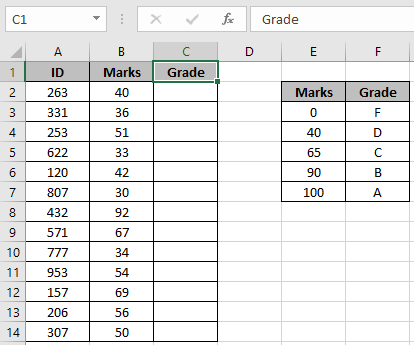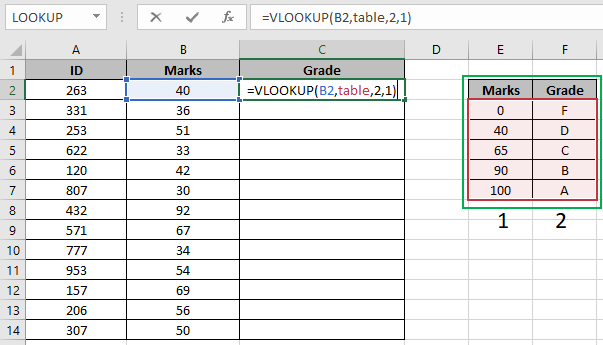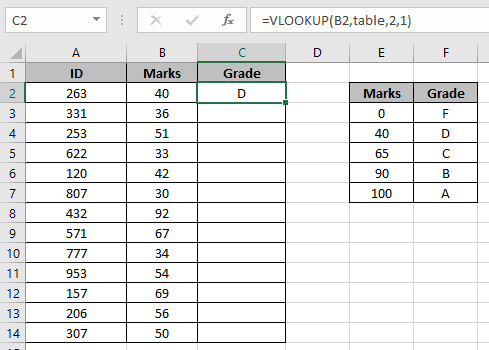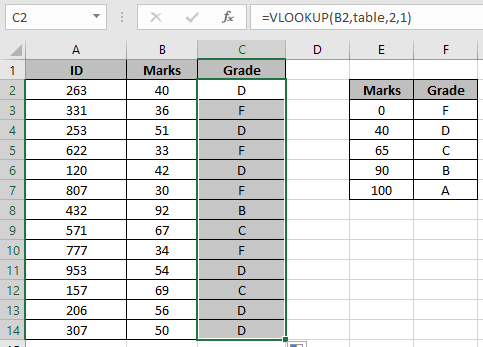# VLOOKUP function to calculate grade in excel

First we should understand a little about, What is VLOOKUP function?
VLOOKUP function looks up the lookup_value in the table_array in first column of a table and returns the corresponding value from the Col_index_num.
Syntax of VLOOKUP function:

 = VLOOKUP ( lookup_value, table_array , col_index_number, [ range_lookup ] )

In VLOOKUP approximate match, if a value is not found then the last value that is less then lookup value is matched and value from given column index is returned.

 = VLOOKUP ( score, table, grade_column, 1 )

VLOOKUP by default does approximate match, if we omit range lookup variable. An approximate match is useful when you want to do an approximate match and when you have your table array sorted in ascending order.

Vlookup looks for the value and if it doesn’t find the value in table array then it matches the value that is less than that value in table array. Lets understand it by an Example.

Let’s understand this function using it in an example.

Here we have Student ID and their respective marks in a test. Now we had to get the grades for each student via looking up in the grade system table.To do so, we will use an attribute of VLOOKUP function which is if VLOOKUP function doesn't find the exact match it looks for the approx match in the table only and only if the last argument of the function is either TRUE or 1.
The marks/score table must be ascending order
Use the formula:

 = VLOOKUP ( B2 , table , 2 , 1 )

How it works?
The Vlookup function looks up the value 40 in the marks column and returns the corresponding value, if matched. If not matches then the function looks for the lesser value than the lookup value (i.e 40) and returns its result.Here the table_array is named range as table in formula and B2 is given as cell reference.As you can see we got the grade for the first student. Now to get all other Grades, we will use shortcut Ctrl + D or use drag down cell option in excel.Here are all the Grades of the class using VLOOKUP function. Below are some of the observational results using the formula.
Notes:

1. Marks / scores table column must be in ascending order or else function can give the wrong result.
2. The VLOOKUP function looks up for the value in the first row of Table_array and extracts corresponding values only right of the lookup_range.
3. The VLOOKUP function last argument of the function must be set to either TRUE or 1 to get the approximate match.
4. The VLOOKUP function returns error if workbook address is invalid or wrong.
5. The VLOOKUP function returns an error if the value is not matched.

Hope you understood how to use the VLOOKUP with Different Excel workbook in Excel. Explore more articles on Excel HLOOKUP and LOOKUP function here. Please feel free to state your query or feedback for the above article.

Related Articles
How to use the VLOOKUP function in Excel: Look up the value in a vertical format table and returns the required result
How to use the HLOOKUP function in Excel: Looks up the value in a horizontal format table and returns the required result
How to VLOOKUP from Different Excel Sheet: Looks up the value in different excel sheet table.